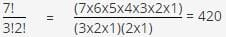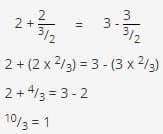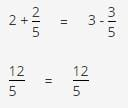Test: Problem Solving- 4

# Test: Problem Solving- 4

Test Description

## 10 Questions MCQ Test General Test Preparation for CUET | Test: Problem Solving- 4

Test: Problem Solving- 4 for SSC CGL 2023 is part of General Test Preparation for CUET preparation. The Test: Problem Solving- 4 questions and answers have been prepared according to the SSC CGL exam syllabus.The Test: Problem Solving- 4 MCQs are made for SSC CGL 2023 Exam. Find important definitions, questions, notes, meanings, examples, exercises, MCQs and online tests for Test: Problem Solving- 4 below.
Solutions of Test: Problem Solving- 4 questions in English are available as part of our General Test Preparation for CUET for SSC CGL & Test: Problem Solving- 4 solutions in Hindi for General Test Preparation for CUET course. Download more important topics, notes, lectures and mock test series for SSC CGL Exam by signing up for free. Attempt Test: Problem Solving- 4 | 10 questions in 20 minutes | Mock test for SSC CGL preparation | Free important questions MCQ to study General Test Preparation for CUET for SSC CGL Exam | Download free PDF with solutions
 1 Crore+ students have signed up on EduRev. Have you?
Test: Problem Solving- 4 - Question 1

### A code is to be made by arranging 7 letters. Three of the letters used will be the letter A, two of the letters used will be the letter B, one of the letters used will be the letter C, and one of the letters used will be the letter D. If there is only one way to present each letter, how many different codes are possible?

Detailed Solution for Test: Problem Solving- 4 - Question 1

Step 1: Analyze the Question :- We have to make a seven-letter code, but some of our letters are repeated. We have three As two Bs, one C, and one D. We have to calculate the possible number of different codes.

Step 2: State the Task :- We'll calculate the number of permutations, remembering to take the repeated letters into account.

Step 3: Approach Strategically :- To calculate the number of permutations where some of the elements are indistinguishable, we'll divide the total number of permutations by the factorial of the number of indistinguishable elements.

So we have :-Test: Problem Solving- 4 - Question 2

### If a rectangular billboard has an area of 104 square feet and a perimeter of 42 feet, what is the length of each of the shorter sides?

Detailed Solution for Test: Problem Solving- 4 - Question 2

Step 1: Analyze the Question :- We know two facts about the rectangle: the area and the perimeter. We need to find the length of the shorter side, so we should try to use the area and perimeter information to set up equations that we can use to solve for the side lengths.
Notice that since the answer choices are all numbers, we can also Backsolve to find the length of the sides.

Step 2:- State the Task Use the definitions of the area and perimeter of the rectangle to solve for the length of each of the two equal shorter sides of the billboard.

Step 3:- Approach Strategically
The area is the product of the two sides, and the perimeter is the sum of the four sides. If L and W are the length and the width of the rectangle, then the area of the rectangle is LW, so LW = 104.

The perimeter of the rectangle is 2(L + W), so 2(L + W) = 42. We can simplify the perimeter equation if we divide both sides by 2, giving us L + W = 21. Because we have two equations and two variables, we can solve for L and W.
The two equations are LW = 104 and L + W = 21.

We are looking for two numbers whose product is 104 and whose sum is 21. We can factor the 104 to determine the pairs of numbers that must sum to 21.

The factors of 104 are 2 x 52, 4 x 26, and 8 x 13.
The only pair whose sum equals 21 is 8 and 13. So L = 13, and W = 8. Choice (C) is correct.
Now, here's the Backsolving solution.
Let's say that you started with (D). If the shorter side is 13, then the two short sides of the billboard total 26 feet. That leaves 42 - 26, or 16 feet for the two longer sides. They must be, therefore, 8 feet each. Except, of course, that is 13 is the "shorter" side, 8 can't be the "longer" side. So, the shorter side must definitely be less than 13 feet; (d) and (E) can be elminated.
Now we test (B). If the shorter side is 7, then the two short sides of the billboard total 14 feet. That leaves 42 - 14, or 28 feet for the two longer sides. They must be, therefore, 14 feet each. Is the area 104? No, as 14 x 7 = 98. Eliminate (B). Even if you don't see why the shorter side has to be longer and decide that you needed to test another choice, the fact that you already know that 8 x 13 yields a valid perimeter would lead you to test (C). And since 8 x 13 = 104, the shorter side is indeed 8. Answer choice (C) is correct.
Step 4: Confirm Your Answer:- Read back over the problem, confirming that your solution accurately follows the information in the question. For example, if you accidentally solved for the longer side, you'd have chosen (D); this step would allow you to change a wrong answer into a right one.

Test: Problem Solving- 4 - Question 3

### If x is an integer and 2.134 x 10x is less than 210,000, what is the greatest possible value for x?

Detailed Solution for Test: Problem Solving- 4 - Question 3

Step 1: Analyze the Question
We know two things about x: it's an integer, and 2.134 x 10% < 210,000.

Find the greatest possible value for X.

Step 3: Approach Strategically
To multiply a decimal quantity by 10%, move the decimal point x places to the right. For 2.134, moving the decimal point 5 places to the right results in the number 213,400. That's just over the limit of 210,000, so the maximum value of x is 4. The correct answer is (D).

A great way to confirm the answer on this type of question is to write the number down on your noteboard before counting the number of decimal places to move.

Test: Problem Solving- 4 - Question 4

John spent 40 percent of his earnings last month on rent and 30 percent less than what he spent on rent to purchase a new dishwasher. What percent of last month's earnings did John have left over?

Detailed Solution for Test: Problem Solving- 4 - Question 4

Step 1: Analyze the Question
John spends 40% on one thing and 30% less than that on another. Since the answer choices are percents, picking 100 is a good idea. Some answer choices are widely spread out. When choices are spread out, estimation and logic are also great approaches.

What percent of last month's earnings did John have left over? We now know that we'd pick \$100 as his earnings. (We care much more about picking manageable numbers than about giving imaginary people a living wage.) It's also important to focus on the fact that we are solving for what he has left after paying for rent and the dishwasher, not what he spent on those things.

Step 3: Approach Strategically
Some answer choices could be logically eliminated right away. After spending 40% of his earnings on rent, he'd have 60% left. Then he spends some more. Therefore, no answer 60% or greater could be possible. That eliminates (D) and (E) very quickly. And since simple combinations of percents are rarely the right answer, the odds of the right answer being “subtract 40% and then subtract 30%" are very small. That makes 100% - 40% - 30% = 30%, choice (A), a safe elimination as well. We could make a 50/50 guess very quickly on this problem, which is sometimes a good thing to do if you are falling behind pace. But let's say that you had the time to solve. Picking \$100 for his earnings, we see that John spends \$40 on rent. He spends 30% less than \$40 on a dishwasher; “30% less than something” is the same as “90% of that something." So John spent 0.7(\$40), or \$28, on a dishwasher. Taking \$40 and \$28, or \$68 away from \$100, John is left with \$32. That's 32% of his original earnings, so choice (B) is correct.

If you misread “30% less than his rent" as "30% of his earnings," and chosen (A) as a result, this step would save you from a wrong answer. Also, (D) is another trap answer that represents the total percentage of this earnings that John spent.

Test: Problem Solving- 4 - Question 5

If negative integers k and p are NOT both even, which of the following must be odd?

Detailed Solution for Test: Problem Solving- 4 - Question 5

Step 1: Analyze the Question
For this abstract number properties question, we can either apply the rules for odd and even numbers directly or simply pick some numbers to solve the question.

We must determine which answer choice must always be odd or, in other words, eliminate any answer choices that can be even.

Step 3: Approach Strategically
For some number properties questions, using rules (if you are certain of them) is faster than Picking Numbers. In this question, the condition that k and p are negative and are "not both even" complicates Picking Numbers but not applying rules. Since we have rules for odd and even numbers, we can apply them directly to the answer choices. We start with (E), since this is a "which of the following" question. (E): Because 2 times any value is even, 2(k + p) will always be even. Subtracting one from an even number will always result in an odd number. Therefore, (E) is always odd and must be the correct answer.

You can confirm your answer by noting that (A) through (D) could be even, judging by odd and even rules. (B) is always even, for example. (A) is odd when k and p are both odd, but the question stem allows for the possibility that one of them is even, and in such a case kp is even.

Test: Problem Solving- 4 - Question 6

Peter read P books last year, and Nikki read N books last year. If Peter read 35 more books than Nikki last year, which of the following reflects the relationship?

Detailed Solution for Test: Problem Solving- 4 - Question 6

Step 1: Analyze the Question
The sentences in this word problem need to be translated into algebraic statements so that we can determine the relationship between the number of books that Peter and Nikki have read.

Once the word problem has been translated, we will apply basic algebra to simplify the statement to match the correct answer choice.

Step 3: Approach Strategically
Translating the phrase "Peter read 35 more books than Nikki," we have P = N + 35.

This translation directly matches (E), but be careful to check that the variables are in the correct order.

Test: Problem Solving- 4 - Question 7

If 2 is the remainder when m is divided by 5, what is the remainder when 3m is divided by 5?

Detailed Solution for Test: Problem Solving- 4 - Question 7

Step 1: Analyze the Question
This question tests our ability to think critically about the characteristics of remainders in division We are told that some number, m, has a remainder of 2 when divided by 5.

We can use our knowledge of number properties to take a particularly strategic approach to this problem. The key will be to pick simple, permissible numbers and apply them to the problem in the question stem.

Step 3: Approach Strategically
Ask yourself what numbers would be permissible for m. Since m has a remainder of 2 when divided by 5, m could be any number 2 greater than a multiple of 5. The simplest number to substitute for m is 7. We know that 5 goes into 7 one time with a remainder of 2. Now, apply 7 to the rest of the question stem: 3 m divided by 5. Well, 3 x 7 = 21, and 21 divided by 5 would leave a remainder of 1. That's (B). Picking Numbers is the most efficient approach to this common GMAT question type.

To double-check your work, you could test any other permissible number for m: 12, 17, 22, etc. If you tried 12, you would find that 3 x 12 = 36 and 36 divided by 5 leaves a remainder of 1. This confirms that (B) is the correct choice.

Test: Problem Solving- 4 - Question 8

If 2 + 2/x = 3 - 3/x , then x =

Detailed Solution for Test: Problem Solving- 4 - Question 8

Step 1: Analyze the Question
This question gives us a complicated-looking equation with one variable. The answer choices are just numbers.

Our task is to solve for the value of x

Step 3: Approach Strategically
Since the answer choices are potential values for the variable in the equation, we could just plug those values back in to see which value makes the equation true. Backsolving is an option whenever you can manageably plug an answer choice into the question stem. Let's say that you started with (D), x = 3/2 That makes the equation:That's not a true statement. So we need to try other values. It's very hard to see whether you needed a larger or smaller x, so it's perfectly fine to try different answer choices. (C) is a sensible choice to test next, as it's the most manageable. It would leave you with 2 + 2 = 3 - 3, which is also false. (E) is the next most manageable. Plugging 5 in for x makes the equationReread the original equation, making sure you didn't make a careless error such as switching the plus and minus signs

Test: Problem Solving- 4 - Question 9

To fill and art exhibit, the students in an art course are assigned to create one epiece of artwork each in the following distribution: 1⁄3 are sculptures, 1⁄8 are oil paintings, 1⁄2 are watercolors and the remaining 10 pieces are mosaics. How many students are in the art class?

Detailed Solution for Test: Problem Solving- 4 - Question 9

Step 1: Analyze the Question
In this question, we are presented with a series of parts that make up the whole- in this case, the number of students in an art class. Notice that most of the whole is identified as fractions of the whole, while one part is identified as a specific quantity. We can use this to our advantage.

Determine the sum of the fractions in the question stem (sculptures, oil paintings, watercolors), since this makes up all but one part of the whole number of students. Subtracting this fraction from 1 will provide the fraction of the whole that is the remaining part (mosaics). Finally, we will solve for the number of students in the class. Note that this question can also be solved by Backsolving, since all of the answer choices are numbers and we can test out the answer choices to see if 10 pieces remain after calculating the number of other pieces of artwork.

Step 3: Approach Strategically
We are told that 1/3 of the students create sculptures, 1/8 create oil paintings, 1/2 create watercolors, and the remaining 10 pieces are mosaics. Let's assume that the total number of students in the class is x. Then the sum of the sculptures, oil paintings, and watercolors is as follows:

(1/3)x + (1/8)x + (1/2)x = x(1/3 + 1/8 + 1/2) = x(8/24 + 3/24 + 12/24) = x(23/24

So the sum of the sculptures, oil paintings, and watercolors is 23/24 of the art in the exhibit. Therefore. the remaining 10 pieces must be 1-23/24 = 1/24 of the total pieces. We can set up the equation:

(1/24)x = 10; x= 240

Choice (C) is correct.

Plug your value for x into the original equation to confirm your calculations are correct.

Test: Problem Solving- 4 - Question 10

A computer company's featured laptop cost \$800 last year. This year, the laptop sold for 15% less than it did last year. Next year, after updates are made to the model, there will be a 25% price increase over this year's price. What will be the price next year?

Detailed Solution for Test: Problem Solving- 4 - Question 10

First, calculate the price of the laptop this year. Then, use that price to determine what the price will be next year. After a 15% decrease in price, the system would sell for 85% of \$800: 0.85 x \$800 = \$680. If there is a 25% increase next year, the system would sell for 125% of this year's price. That would be 125% of \$680: 1.25 x \$680 = \$850 (D).

## General Test Preparation for CUET

90 videos|175 docs|620 tests
 Use Code STAYHOME200 and get INR 200 additional OFF Use Coupon Code
Information about Test: Problem Solving- 4 Page
In this test you can find the Exam questions for Test: Problem Solving- 4 solved & explained in the simplest way possible. Besides giving Questions and answers for Test: Problem Solving- 4, EduRev gives you an ample number of Online tests for practice

## General Test Preparation for CUET

90 videos|175 docs|620 tests